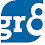# Simple Interest and Compound Interest Difference

I need money. I don't have enough to meet my requirement. Then what should I Do?

Well I have 3 wonderful options.
1. Robing a Bank : It needs Courage, Effort, Planning and Hard-work. Well I am sure that I don't have any of these.
2. To kidnap you and ask your dad for money : Ummmm, not a good idea!!! Nexxxxxxxt
3. To borrow the money from my friend. Sounds good, isn't it?
• But Nobody gonna give me money if Isay that I'm not going to return it to him. So, I should promise him that I will return the amount within a specif amount of time (not really :P). And in-order to TEMPT him, I also will promise him that I will return his money with some ADDITIONAL money. This additional money is called INTEREST.
• There are TWO types of Interests are there
When money is borrowed at Simple Interest, the interest charged is same irrespective of the period involved.
I mean, if Simple Interest for One year is Rs 1000/-, then for 2 Years with teh same rate percent, it will be 2X1000 = 2000/-

So, for 8 years it will be 8 X1000 = 8000.

So, if the Simple Interest (or SI in shorter form) on a certain sum is Rs 600 in 3 yrs, then the SI on that sum for one year will be 600/3 = 200

Now have a look at some formulas :

SI = (P*T*R)/100

Where
• SI=Simple Interest
• P= Principle (the actual money borrowed)
• T=Time  in Years
• R=Rate of % per annum (The percentage of the Principle, we should pay as the Interest)
So, Obviously

• P = (100I) /TR
• T = (100I) /PR
• R = (100I) / PT
Now, how much money we should pay to the Lender?
Its the total of the money we have taken from him and the money we should pay in the name of Interest.

So, Amount A = P+I       The actual amount (Principle) + Interest

Now lets see some Examples

1.nice explanation mam . . . A marvelous site for studnts .

2.i ve got interest in apps solving after ur easy explanations in tis website.. thnx a lot :)

3.4.Mam first of all i appreciate your works to help us.Nice work.I just wanted to have an idea regarding what kind of awareness area we have to prepare for UIIC AO Exam...It would be great help .....Thankz

1.If you are asking about Financial Awareness, then you should prepare basic financial terminology and last six months' economic events Baruah. Thats enough. All the best :)

5.Interesting explanations making very easier to understand.........

6.way of explanation is so nice.